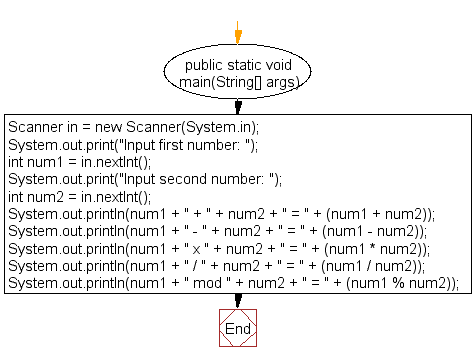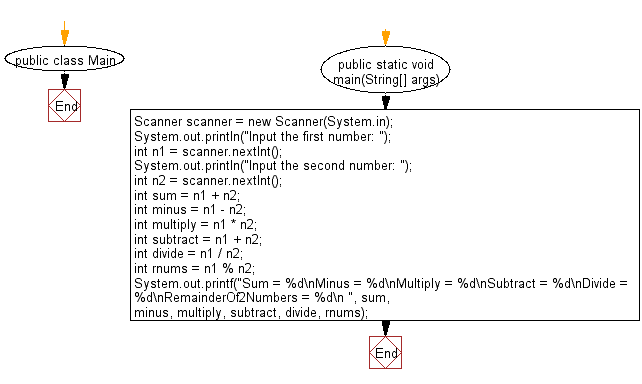﻿ Java: Print the sum, multiply, subtract, divide of 2 numbers# Java Exercises: Print the sum, multiply, subtract, divide and remainder of two numbers

## Java Basic: Exercise-6 with Solution

Write a Java program to print the sum (addition), multiply, subtract, divide and remainder of two numbers.

Test Data:
Input first number: 125
Input second number: 24

Pictorial Presentation:Sample Solution:

Java Code:

``````import java.util.Scanner;

public class Exercise6 {

public static void main(String[] args) {
Scanner in = new Scanner(System.in);

System.out.print("Input first number: ");
int num1 = in.nextInt();

System.out.print("Input second number: ");
int num2 = in.nextInt();

System.out.println(num1 + " + " + num2 + " = " +
(num1 + num2));

System.out.println(num1 + " - " + num2 + " = " +
(num1 - num2));

System.out.println(num1 + " x " + num2 + " = " +
(num1 * num2));

System.out.println(num1 + " / " + num2 + " = " +
(num1 / num2));

System.out.println(num1 + " mod " + num2 + " = " +
(num1 % num2));
}

}
```
```

Sample Output:

```Input first number: 125
Input second number: 24
125 + 24 = 149
125 - 24 = 101
125 x 24 = 3000
125 / 24 = 5
125 mod 24 = 5
```

Flowchart:Sample Solution:

Java Code:

``````import java.util.Scanner;
public class Main {
public static void main(String[] args) {
Scanner scanner = new Scanner(System.in);
System.out.println("Input the first number: ");
int n1 = scanner.nextInt();
System.out.println("Input the second number: ");
int n2 = scanner.nextInt();
int sum = n1 + n2;
int minus = n1 - n2;
int multiply = n1 * n2;
int subtract = n1 + n2;
int divide = n1 / n2;
int rnums = n1 % n2;
System.out.printf("Sum = %d\nMinus = %d\nMultiply = %d\nSubtract = %d\nDivide = %d\nRemainderOf2Numbers = %d\n ", sum, minus, multiply, subtract, divide, rnums);
}
}
```
```

Sample Output:

```Input the first number:
6
Input the second number:
5
Sum = 11
Minus = 1
Multiply = 30
Subtract = 11
Divide = 1
RemainderOf2Numbers = 1
```

Flowchart:Java Code Editor:

What is the difficulty level of this exercise?

Test your Programming skills with w3resource's quiz.

﻿

## Java: Tips of the Day

countOccurrences

Counts the occurrences of a value in an array.

Use Arrays.stream().filter().count() to count total number of values that equals the specified value.

```public static long countOccurrences(int[] numbers, int value) {
return Arrays.stream(numbers)
.filter(number -> number == value)
.count();
}
```

Ref: https://bit.ly/3kCAgLb